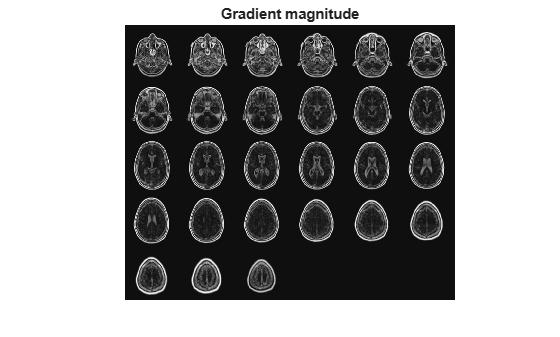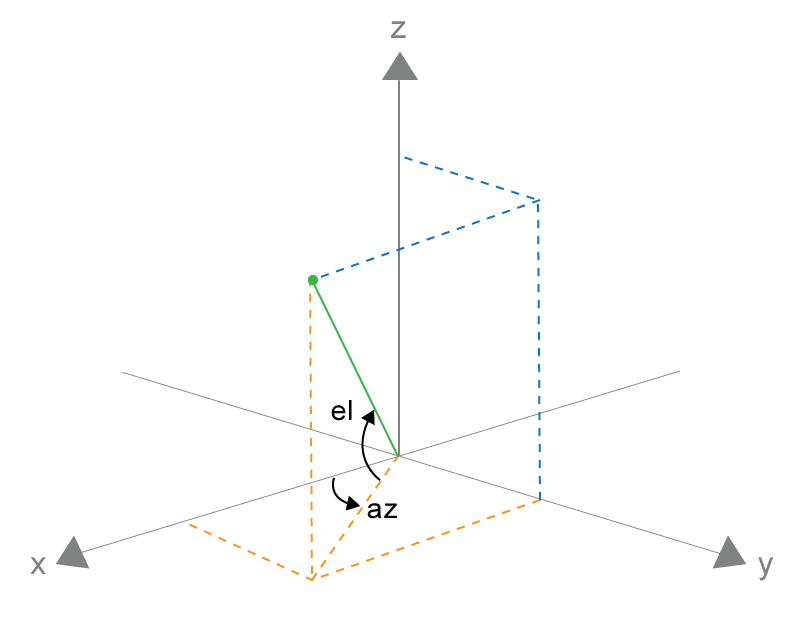Find gradient magnitude and direction of 3-D image

Description

example

[Gmag,Gazimuth,Gelevation] = imgradient3(I) returns the gradient magnitude, Gmag, gradient direction, Gazimuth, and gradient elevation Gelevation of the 3-D grayscale or binary image I.

[Gmag,Gazimuth,Gelevation] = imgradient3(I,method) calculates the gradient magnitude, direction, and elevation using the specified method.

[Gmag,Gazimuth,Gelevation] = imgradient3(Gx,Gy,Gz) calculates the gradient magnitude, direction, and elevation from the directional gradients Gx, Gy, and Gz in the x, y, and z directions, respectively.

Examples

collapse all

Read 3-D data into the workspace and prepare it for processing.

sz = volData.siz;
vol = squeeze(volData.D);

Visualize the gradient magnitude as a montage.

figure,
montage(reshape(Gmag,sz(1),sz(2),1,sz(3)),'DisplayRange',[])Input Arguments

collapse all

Input image, specified as a 3-D grayscale image or 3-D binary image.

Data Types: single | double | int8 | int16 | int32 | int64 | uint8 | uint16 | uint32 | uint64 | logical

Gradient operator, specified as one of the following values.

Value

Meaning

'sobel'

Sobel gradient operator. The gradient of a pixel is a weighted sum of pixels in the 3-by-3-by-3 neighborhood. For example, in the depth (z) direction, the weights in the three planes are:

plane z-1plane zplane z+1
[ 1  3  1
3  6  3
1  3  1 ]
[ 0  0  0
0  0  0
0  0  0 ]
[ -1  -3  -1
-3  -6  -3
-1  -3  -1 ]

'prewitt'

Prewitt gradient operator. The gradient of a pixel is a weighted sum of pixels in the 3-by-3-by-3 neighborhood. For example, in the depth (z) direction, the weights in the three planes are:

plane z-1plane zplane z+1
[ 1  1  1
1  1  1
1  1  1 ]
[ 0  0  0
0  0  0
0  0  0 ]
[ -1  -1  -1
-1  -1  -1
-1  -1  -1 ]

'central'

Central difference gradient. The gradient of a pixel is a weighted difference of neighboring pixels. For example, in the depth (z) direction, dI/dz = (I(z+1) - I(z-1))/2.

'intermediate'

Intermediate difference gradient. The gradient of a pixel is the difference between an adjacent pixel and the current pixel. For example, in the depth (z) direction, dI/dz = I(z+1) - I(z).

When applying the gradient operator at the boundaries of the image, imgradient3 assumes values outside the bounds of the image equal the nearest image border value. This behavior is similar to the 'replicate' boundary option in imfilter.

Data Types: char | string

Horizontal gradient, specified as a 3-D numeric array. The horizontal (x) axis points in the direction of increasing column subscripts. You can use the imgradientxyz function to calculate Gx.

Data Types: single | double | int8 | int16 | int32 | int64 | uint8 | uint16 | uint32 | uint64

Vertical gradient, specified as a 3-D numeric array of the same size as Gx. The vertical (y) axis points in the direction of increasing row subscripts. You can use the imgradientxyz function to calculate Gy.

Data Types: single | double | int8 | int16 | int32 | int64 | uint8 | uint16 | uint32 | uint64

Depth gradient, specified as a 3-D numeric array of the same size as Gx. The depth (z) axis points in the direction of increasing plane subscripts. You can use the imgradientxyz function to calculate Gz.

Data Types: single | double | int8 | int16 | int32 | int64 | uint8 | uint16 | uint32 | uint64

Output Arguments

collapse all

Magnitude of the gradient vector, returned as a 3-D numeric array of the same size as image I or the directional gradients, Gx, Gy, and Gz.

Gmag is of class double, unless the input image or any of the directional gradients are of class single. In this case, Gmag is of class single.

Azimuthal angle, returned as a 3-D numeric array of the same size as the gradient magnitude, Gmag. Gazimuth contains angles in degrees within the range [-180, 180] measured between positive x-axis and the projection of the point on the x-y plane.

Gazimuth is of class double, unless the input image or any of the directional gradients are of class single. In this case, Gmag is of class single.

Gazimuth and GelevationGradient elevation, returned as a 3-D numeric array of the same size as the gradient magnitude, Gmag. Gelevation contains angles in degrees within the range [-90, 90] measured between the radial line and the x-y plane.

Gelevation is of class double, unless the input image or any of the directional gradients are of class single. In this case, Gmag is of class single.

Algorithms

imgradient3 does not normalize the gradient output. If the range of the gradient output image has to match the range of the input image, consider normalizing the gradient image, depending on the method argument used. For example, with a Sobel kernel, the normalization factor is 1/44 and for Prewitt, the normalization factor is 1/18.